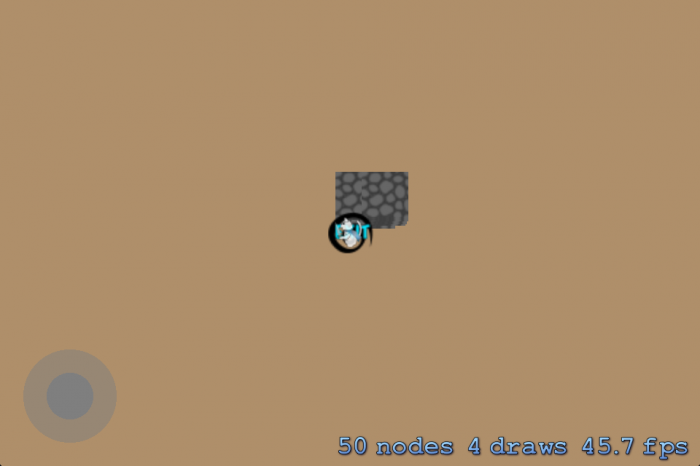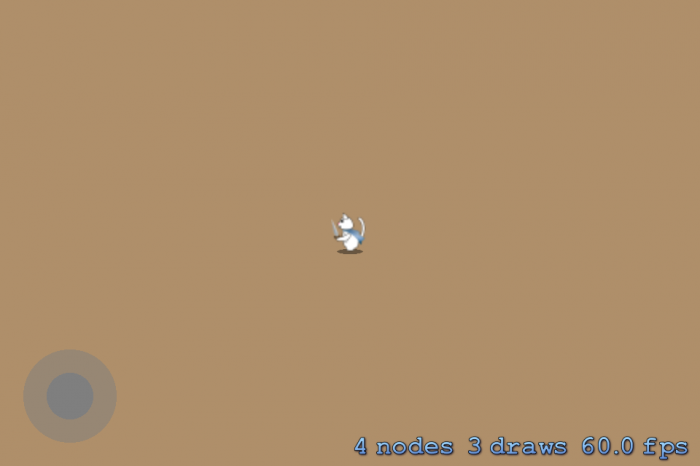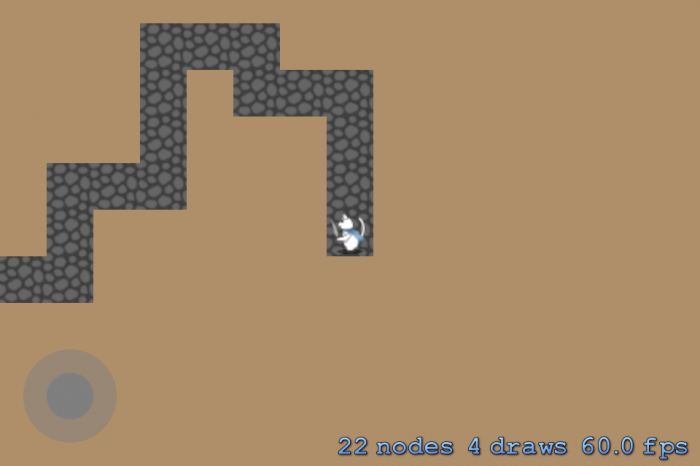# Procedural Level Generation in Games Tutorial: Part 1

A tutorial on procedural level generation using the Drunkard Walk algorithm. By Kim Pedersen.

Leave a rating/review
Save for later
Share

45 mins

## Generating the Floor

You're going to place ground or floor tiles procedurally in the map using the Drunkard Walk algorithm discussed above. In Map.m, you already implemented part of the algorithm so that it finds a random start position (step 1) and loops a desired number of times (step 4). Now you need to implement steps 2 and 3 to generate the actual floor tiles within the loop you created.

To make the `Map` class a bit more flexible, you'll start by adding a dedicated method to generate a procedural map. This will also be handy if you later need to regenerate the map.

Open Map.h and add the following method declaration to the interface:

```- (void) generate;
```

In Map.m, add the following import to the top of the file:

```#import "MapTiles.h"
```

Add the following code right above the `@implementation` line:

```@interface Map ()
@property (nonatomic) MapTiles *tiles;
@end
```

The class extension holds one private property, which is a pointer to a `MapTiles` object. You'll use this object for easy grid handling in the map generation. You're keeping it private since you don't want to change the `MapTiles` object from outside the `Map` class.

Next, implement the `generate` method in Map.m:

```- (void) generate
{
self.tiles = [[MapTiles alloc] initWithGridSize:self.gridSize];
[self generateTileGrid];
}
```

First the method allocates and initializes a `MapTiles` object, then it generates a new tile grid by calling `generateTileGrid`.

In Map.m, go to `initWithGridSize:` and delete this line:

```[self generateTileGrid];
```

You deleted that line because map generation should no longer occur immediately when you create a `Map` object.

It's time to add the code to generate the floor of the dungeon. Do you remember the remaining steps of the Drunkard Walk algorithm? You choose a random direction and then place a floor at the new coordinates.

The first step is to add a convenience method to provide a random number between two values. Add the following method in Map.m:

```- (NSInteger) randomNumberBetweenMin:(NSInteger)min andMax:(NSInteger)max
{
return min + arc4random() % (max - min);
}
```

You'll use this method to return a random number between min and max, both inclusive.

Return to `generateTileGrid` and replace its contents with the following:

```CGPoint startPoint = CGPointMake(self.tiles.gridSize.width / 2, self.tiles.gridSize.height / 2);
// 1
[self.tiles setTileType:MapTileTypeFloor at:startPoint];
NSUInteger currentFloorCount = 1;
// 2
CGPoint currentPosition = startPoint;
while ( currentFloorCount < self.maxFloorCount )
{
// 3
NSInteger direction = [self randomNumberBetweenMin:1 andMax:4];
CGPoint newPosition;
// 4
switch ( direction )
{
case 1: // Up
newPosition = CGPointMake(currentPosition.x, currentPosition.y - 1);
break;
case 2: // Down
newPosition = CGPointMake(currentPosition.x, currentPosition.y + 1);
break;
case 3: // Left
newPosition = CGPointMake(currentPosition.x - 1, currentPosition.y);
break;
case 4: // Right
newPosition = CGPointMake(currentPosition.x + 1, currentPosition.y);
break;
}
//5
if([self.tiles isValidTileCoordinateAt:newPosition] &&
![self.tiles isEdgeTileAt:newPosition] &&
[self.tiles tileTypeAt:newPosition] == MapTileTypeNone)
{
currentPosition = newPosition;
[self.tiles setTileType:MapTileTypeFloor at:currentPosition];
currentFloorCount++;
}
}
// 6
_exitPoint = currentPosition;
// 7
NSLog(@"%@", [self.tiles description]);
```

This is what the code is doing:

1. It marks the tile at coordinates `startPoint` in the grid as a floor tile and therefore initializes `currentFloorCount` with a count of 1.
2. `currentPosition` is the current position in the grid. The code initializes it to the `startPoint` coordinates where the Drunkard Walk algorithm will start.
3. Here the code chooses a random number between 1 and 4, providing a direction to move (1 = UP, 2 = DOWN, 3 = LEFT, 4 = RIGHT).
4. Based on the random number chosen in the above step, the code calculates a new position in the grid.
5. If the newly calculated position is valid and not an edge, and does not already contain a tile, this part adds a floor tile at that position and increments `currentFloorCount` by 1.
6. Here the code sets the last tile placed to the exit point. This is the goal of the map.
7. Lastly, the code prints the generated tile grid to the console.

Build and run. The game runs with no visible changes, but it fails to write the tile grid to the console. Why is that?

[spoiler title="Solution"]You never call `generate` on the `Map` class during `MyScene` initialization. Therefore, you created the map object but don't actually generate the tiles.[/spoiler]

To fix this, go to MyScene.m and in `initWithSize:`, replace the line `self.map = [[Map alloc] init]` with the following:

```self.map = [[Map alloc] initWithGridSize:CGSizeMake(48, 48)];
self.map.maxFloorCount = 64;
[self.map generate];
```

This generates a new map with a grid size of 48 by 48 tiles and a desired maximum floor count of 64. Once you set the `maxFloorCount` property, you generate the map.

Build and run again, and you should see an output that resembles something similar to, but probably not exactly like (remember, it's random), the following:HOORAY!! You have generated a procedural level. Pat yourself on the back and get ready to show your masterpiece on the big – or small – screen.

## Converting a Tile Grid into Tiles

Plotting your level in the console is a good way to debug your code but a poor way to impress your player. The next step is to convert the grid into actual tiles.

The starter project already includes a texture atlas containing the tiles. To load the atlas into memory, add a private property to the class extension of Map.m, as well as a property to hold the size of a tile:

```@property (nonatomic) SKTextureAtlas *tileAtlas;
@property (nonatomic) CGFloat tileSize;
```

Initialize these two properties in `initWithGridSize:`, just after setting the value of `_exitPoint`:

```self.tileAtlas = [SKTextureAtlas atlasNamed:@"tiles"];

NSArray *textureNames = [self.tileAtlas textureNames];
SKTexture *tileTexture = [self.tileAtlas textureNamed:(NSString *)[textureNames firstObject]];
self.tileSize = tileTexture.size.width;
```

After loading the texture atlas, the above code reads the texture names from the atlas. It uses the first name in the array to load a texture and stores that texture's width as `tileSize`. This code assumes textures in the atlas are squares (same width and height) and are all of the same size.

Note: Using a texture atlas reduces the number of draw calls necessary to render the map. Every draw call adds overhead to the system because Sprite Kit has to perform extra processing to set up the GPU for each one. By using a single texture atlas, the entire map may be drawn in as few as a single draw call. The exact number will depend on several things, but in this app, those won't come into play. To learn more, check out Chapter 25 in iOS Games by Tutorials, Performance: Texture Atlases.

Still inside Map.m, add the following method:

```- (void) generateTiles
{
// 1
for ( NSInteger y = 0; y < self.tiles.gridSize.height; y++ )
{
for ( NSInteger x = 0; x < self.tiles.gridSize.width; x++ )
{
// 2
CGPoint tileCoordinate = CGPointMake(x, y);
// 3
MapTileType tileType = [self.tiles tileTypeAt:tileCoordinate];
// 4
if ( tileType != MapTileTypeNone )
{
// 5
SKTexture *tileTexture = [self.tileAtlas textureNamed:[NSString stringWithFormat:@"%i", tileType]];
SKSpriteNode *tile = [SKSpriteNode spriteNodeWithTexture:tileTexture];
// 6
tile.position = tileCoordinate;
// 7
}
}
}
}
```

`generateTiles` converts the internal tile grid into actual tiles by:

1. Two `for` loops, one for x and one for y, iterate through each tile in the grid.
2. This converts the current x- and y-values into a `CGPoint` structure for the position of the tile within the grid.
3. Here the code determines the type of tile at this position within the grid.
4. If the tile type is not an empty tile, then the code proceeds with creating the tile.
5. Based on the tile type, the code loads the respective tile texture from the texture atlas and assigns it to a `SKSpriteNode` object. Remember that the tile type (integer) is the same as the file name of the texture, as explained earlier.
6. The code sets the position of the tile to the tile coordinate.
7. Then it adds the created tile node as a child of the map object. This is done to ensure proper scrolling by grouping the tiles to the map where they belong.

Finally, make sure the grid is actually turned into tiles by inserting the following line into the `generate` method in Map.m, after `[self generateTileGrid]`:

```[self generateTiles];
```

Build and run — but the result is not as expected. The game incorrectly places the tiles in a big pile, as illustrated here:The reason is straightforward: When positioning the tile, the current code sets the tile's position to the position within the internal grid and not relative to screen coordinates.

You need a new method to convert grid coordinates into screen coordinates, so add the following to Map.m:

```- (CGPoint) convertMapCoordinateToWorldCoordinate:(CGPoint)mapCoordinate
{
return CGPointMake(mapCoordinate.x * self.tileSize,  (self.tiles.gridSize.height - mapCoordinate.y) * self.tileSize);
}
```

By multiplying the grid (map) coordinate by the tile size, you calculate the horizontal position. The vertical position is slightly more complicated. Remember that the coordinates (0,0) in Sprite Kit represent the bottom-left corner. In the tile grid, the position of (0,0) is the top-left corner (see Figure 2 above). Hence, in order to correctly position the tile, you need to invert its vertical placement. You do this by subtracting the y-position of the tile in the grid by the total height of the grid and multiplying it by the tile size.

Revisit `generateTiles` and change the line that sets `tile.position` to the following:

```tile.position = [self convertMapCoordinateToWorldCoordinate:CGPointMake(tileCoordinate.x, tileCoordinate.y)];
```

Also, change the line that sets `_exitPoint` in `generateTileGrid` to the following:

```_exitPoint = [self convertMapCoordinateToWorldCoordinate:currentPosition];
```

Build and run – oh no, where did the tiles go?Well, they are still there – they're just outside the visible area. You can easily fix this by changing the player's spawn position. You will apply a simple yet effective strategy where you set the spawn point to the position of the `startPoint` in `generateTileGrid`.

Go to `generateTileGrid` and add the following line at the very bottom of the method:

```_spawnPoint = [self convertMapCoordinateToWorldCoordinate:startPoint];
```

The spawn point is the pair of screen coordinates where the game should place the player at the beginning of the level. Hence, you calculate the world coordinates from the grid coordinates.

Build and run, and take the cat for a walk around the procedural world. Maybe you will even find the exit?Try playing around with different grid sizes and max number of floor tiles to see how it affects the map generation.

One obvious issue now is that the cat can stray from the path. And we all know what happens when cats stray, right? All the songbirds of the world shiver. So, time to put up some walls.Kim Pedersen

Kim Pedersen

Author